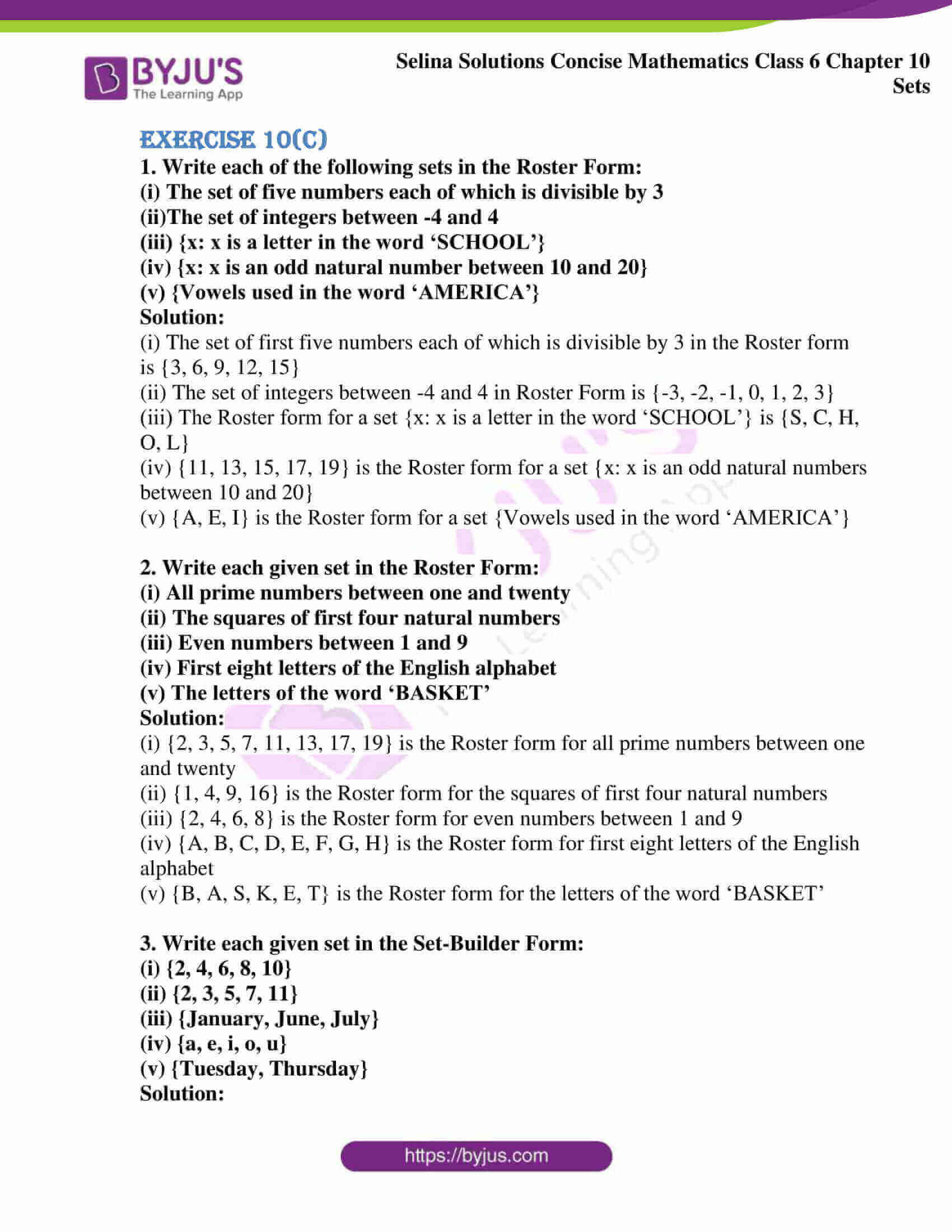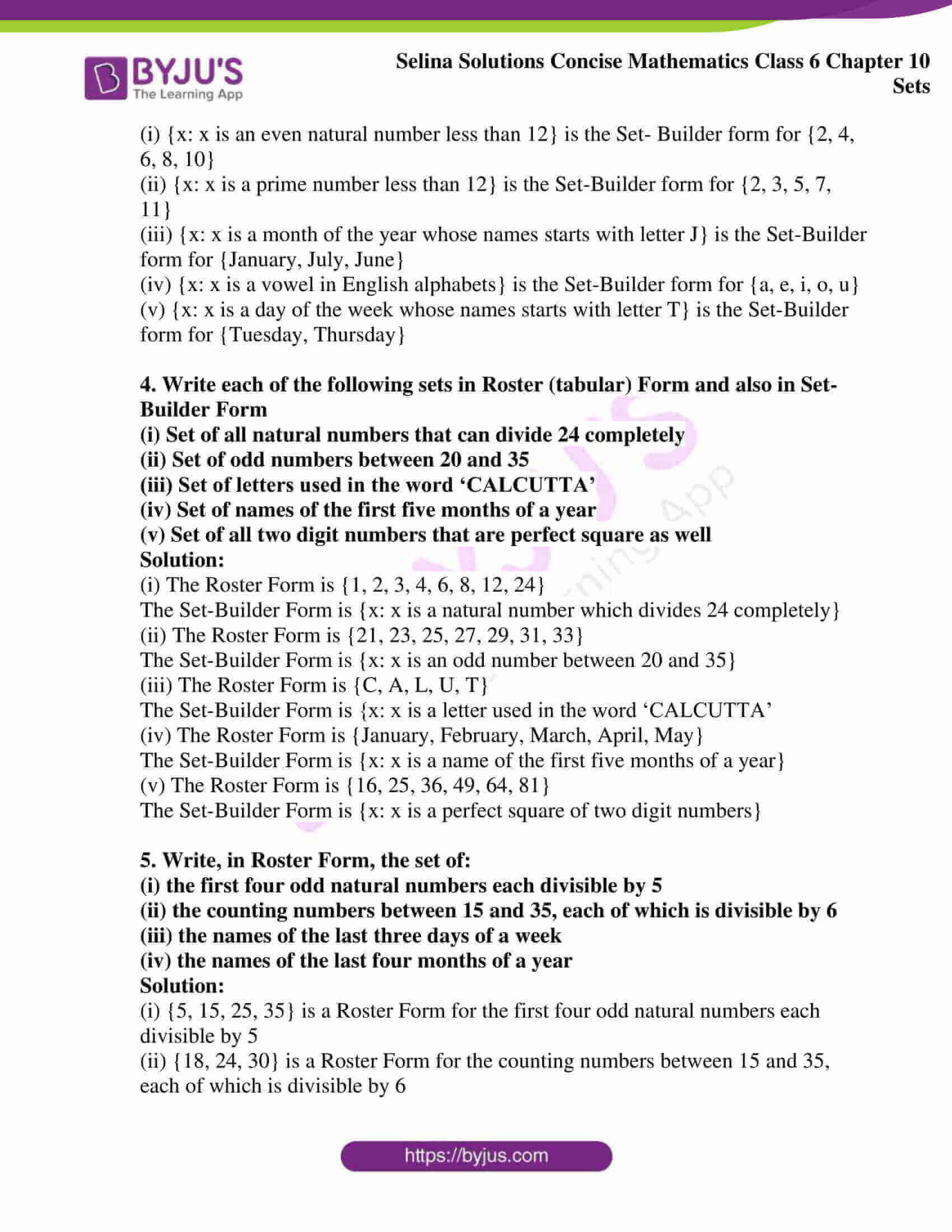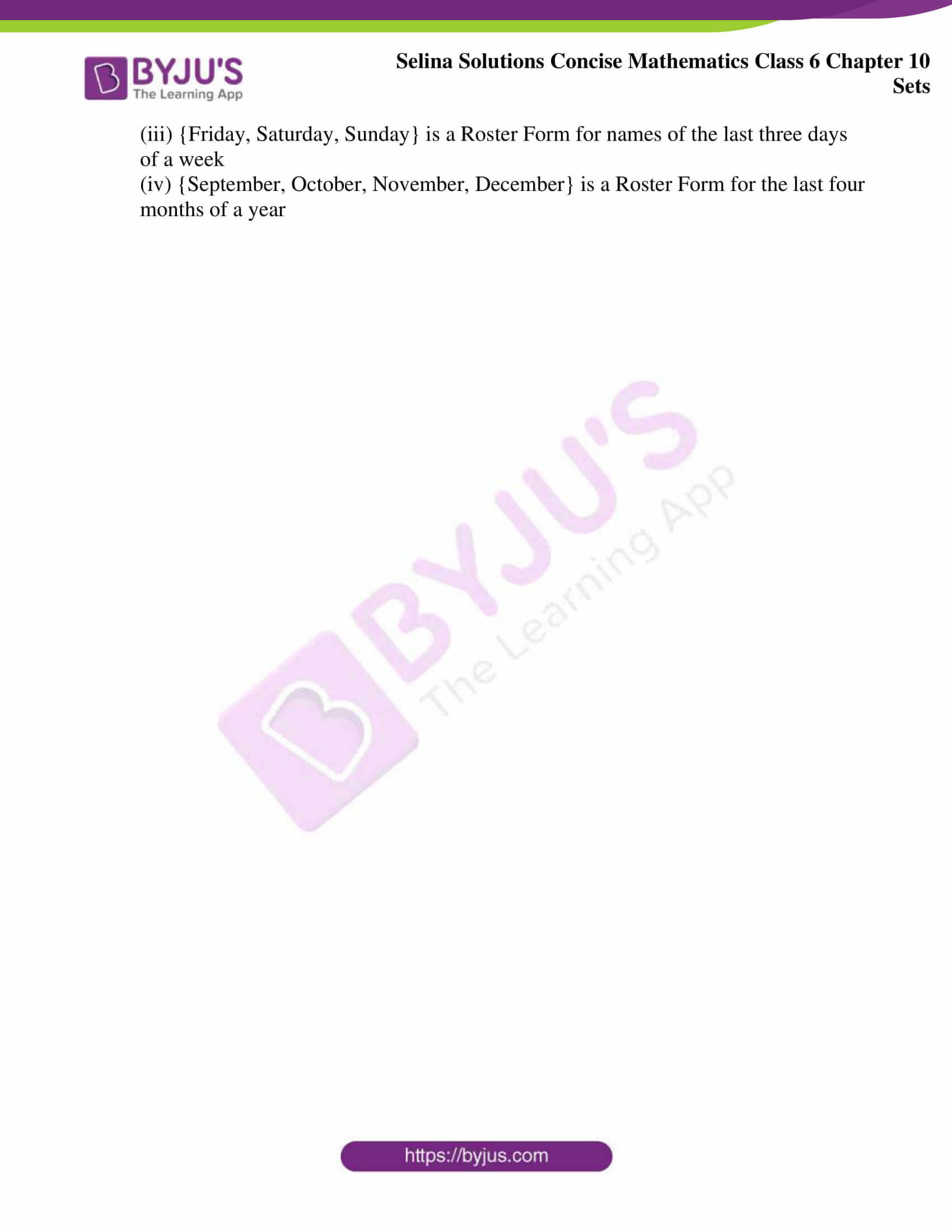# Selina Solutions Concise Mathematics Class 6 Chapter 10: Sets Exercise 10(C)

Selina Solutions Concise Mathematics Class 6 Chapter 10 Sets Exercise 10(C) has accurate answers, which help students to understand the Roster and Set builder method effectively. Students can improve their problem solving abilities by solving textbook questions, using Solutions formulated by expert teachers. These Solutions also help students to understand the method of solving questions in a short duration. Selina Solutions are the key resources in scoring high marks among students. Download PDF format of Selina Solutions Concise Mathematics Class 6 Chapter 10 Sets Exercise 10(C), from the links mentioned below

## Selina Solutions Concise Mathematics Class 6 Chapter 10: Sets Exercise 10(C) Download PDF### Access other exercises of Selina Solutions Concise Mathematics Class 6 Chapter 10 Sets

Exercise 10(A) Solutions

Exercise 10(B) Solutions

Exercise 10(D) Solutions

Exercise 10(E) Solutions

### Access Selina Solutions Concise Mathematics Class 6 Chapter 10: Sets Exercise 10(C)

#### Exercise 10(C)

1. Write each of the following sets in the Roster Form:

(i) The set of five numbers each of which is divisible by 3

(ii)The set of integers between -4 and 4

(iii) {x: x is a letter in the word ‘SCHOOL’}

(iv) {x: x is an odd natural number between 10 and 20}

(v) {Vowels used in the word ‘AMERICA’}

Solution:

(i) The set of first five numbers each of which is divisible by 3 in the Roster form is {3, 6, 9, 12, 15}

(ii) The set of integers between -4 and 4 in Roster Form is {-3, -2, -1, 0, 1, 2, 3}

(iii) The Roster form for a set {x: x is a letter in the word ‘SCHOOL’} is {S, C, H, O, L}

(iv) {11, 13, 15, 17, 19} is the Roster form for a set {x: x is an odd natural numbers between 10 and 20}

(v) {A, E, I} is the Roster form for a set {Vowels used in the word ‘AMERICA’}

2. Write each given set in the Roster Form:

(i) All prime numbers between one and twenty

(ii) The squares of first four natural numbers

(iii) Even numbers between 1 and 9

(iv) First eight letters of the English alphabet

(v) The letters of the word ‘BASKET’

Solution:

(i) {2, 3, 5, 7, 11, 13, 17, 19} is the Roster form for all prime numbers between one and twenty

(ii) {1, 4, 9, 16} is the Roster form for the squares of first four natural numbers

(iii) {2, 4, 6, 8} is the Roster form for even numbers between 1 and 9

(iv) {A, B, C, D, E, F, G, H} is the Roster form for first eight letters of the English alphabet

(v) {B, A, S, K, E, T} is the Roster form for the letters of the word ‘BASKET’

3. Write each given set in the Set-Builder Form:

(i) {2, 4, 6, 8, 10}

(ii) {2, 3, 5, 7, 11}

(iii) {January, June, July}

(iv) {a, e, i, o, u}

(v) {Tuesday, Thursday}

Solution:

(i) {x: x is an even natural number less than 12} is the Set- Builder form for {2, 4, 6, 8, 10}

(ii) {x: x is a prime number less than 12} is the Set-Builder form for {2, 3, 5, 7, 11}

(iii) {x: x is a month of the year whose names starts with letter J} is the Set-Builder form for {January, July, June}

(iv) {x: x is a vowel in English alphabets} is the Set-Builder form for {a, e, i, o, u}

(v) {x: x is a day of the week whose names starts with letter T} is the Set-Builder form for {Tuesday, Thursday}

4. Write each of the following sets in Roster (tabular) Form and also in Set-Builder Form

(i) Set of all natural numbers that can divide 24 completely

(ii) Set of odd numbers between 20 and 35

(iii) Set of letters used in the word ‘CALCUTTA’

(iv) Set of names of the first five months of a year

(v) Set of all two digit numbers that are perfect square as well

Solution:

(i) The Roster Form is {1, 2, 3, 4, 6, 8, 12, 24}

The Set-Builder Form is {x: x is a natural number which divides 24 completely}

(ii) The Roster Form is {21, 23, 25, 27, 29, 31, 33}

The Set-Builder Form is {x: x is an odd number between 20 and 35}

(iii) The Roster Form is {C, A, L, U, T}

The Set-Builder Form is {x: x is a letter used in the word ‘CALCUTTA’

(iv) The Roster Form is {January, February, March, April, May}

The Set-Builder Form is {x: x is a name of the first five months of a year}

(v) The Roster Form is {16, 25, 36, 49, 64, 81}

The Set-Builder Form is {x: x is a perfect square of two digit numbers}

5. Write, in Roster Form, the set of:

(i) the first four odd natural numbers each divisible by 5

(ii) the counting numbers between 15 and 35, each of which is divisible by 6

(iii) the names of the last three days of a week

(iv) the names of the last four months of a year

Solution:

(i) {5, 15, 25, 35} is a Roster Form for the first four odd natural numbers each divisible by 5

(ii) {18, 24, 30} is a Roster Form for the counting numbers between 15 and 35, each of which is divisible by 6

(iii) {Friday, Saturday, Sunday} is a Roster Form for names of the last three days of a week

(iv) {September, October, November, December} is a Roster Form for the last four months of a year